# Details for `oura-hrv-activity-sleep.ipynb`

#### Description

A notebook adapted from Herman de Vries' blogpost and code on using Oura data to explore the links between HRV, physical activity and sleep

#### Tags & Data Sources

hrv heart rate sleep activity Oura Connect

from data from oura ring 2022 on like 7th or 8th cell: Error in runSum(x, n): n = 7 is outside valid range: [1, 6] Traceback: 1. df %>% rename

DG , 1 year, 4 months ago

# Relationship between HRV, Sleep & physical activity in personal Oura ring data.¶

## Background¶

This notebook is based on the work by Herman de Vries, which he published in a very interesting blog post. Herman also presented his work at one of the Open Humans self-research chats. Following the chat Herman was so kind to publish the code he used for analyzing his Oura data as an HTML render of his R code.

If you want to learn more about what Herman learned and how he approached his learning from his Oura data you should definitely read his blog post, as this code is not fully annotated yet.

## This notebook¶

I took Herman's code and copy & pasted most of it right into the Exploratory framework of Open Humans. I only made minor changes to the preamble of the notebook in which the data is being loaded. Herman used a CSV export from Oura, while this notebook makes use of the JSON file of your historic data that's stored in Open Humans.

What this means: If you want to run this notebook right away on your own Oura data you have to import it into Open Humans by going to https://oura.openhumans.org/. Once your data is stored in Open Humans the notebook should run from start to finish without any issues.

## Data import¶

Below is the boring code that loads your personal Oura data and puts it into a format that's in line with Herman's original code. If you're not interested in such things you can straight jump to the next headline.

```── Attaching packages ─────────────────────────────────────── tidyverse 1.3.0 ──

✔ ggplot2 3.3.0     ✔ purrr   0.3.4
✔ tibble  3.0.1     ✔ dplyr   0.8.5
✔ tidyr   1.1.0     ✔ stringr 1.4.0
✔ readr   1.3.1     ✔ forcats 0.5.0

── Conflicts ────────────────────────────────────────── tidyverse_conflicts() ──

Attaching package: ‘jsonlite’

The following object is masked from ‘package:purrr’:

flatten

```
````stat_bin()` using `bins = 30`. Pick better value with `binwidth`.

`stat_bin()` using `bins = 30`. Pick better value with `binwidth`.

```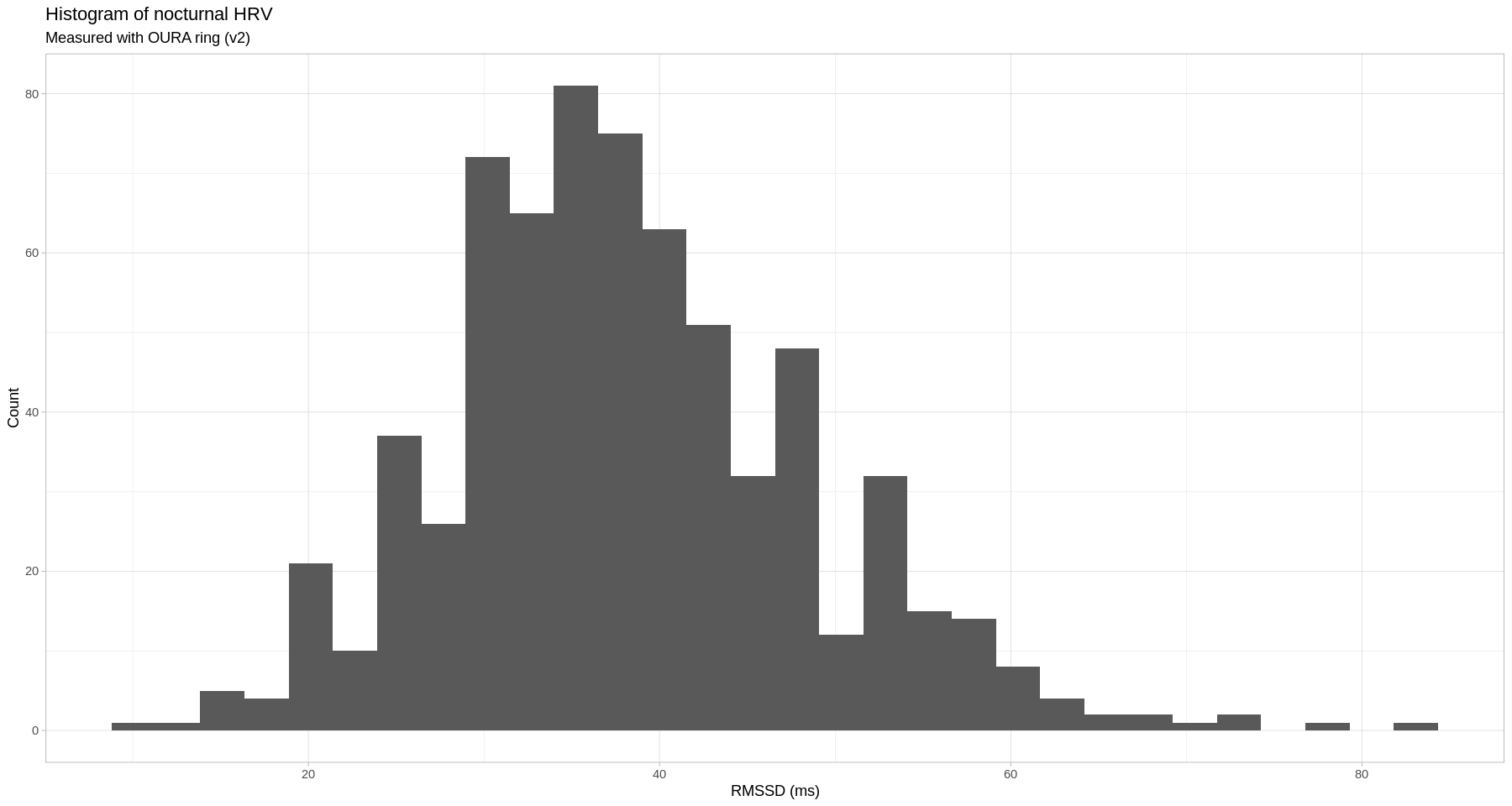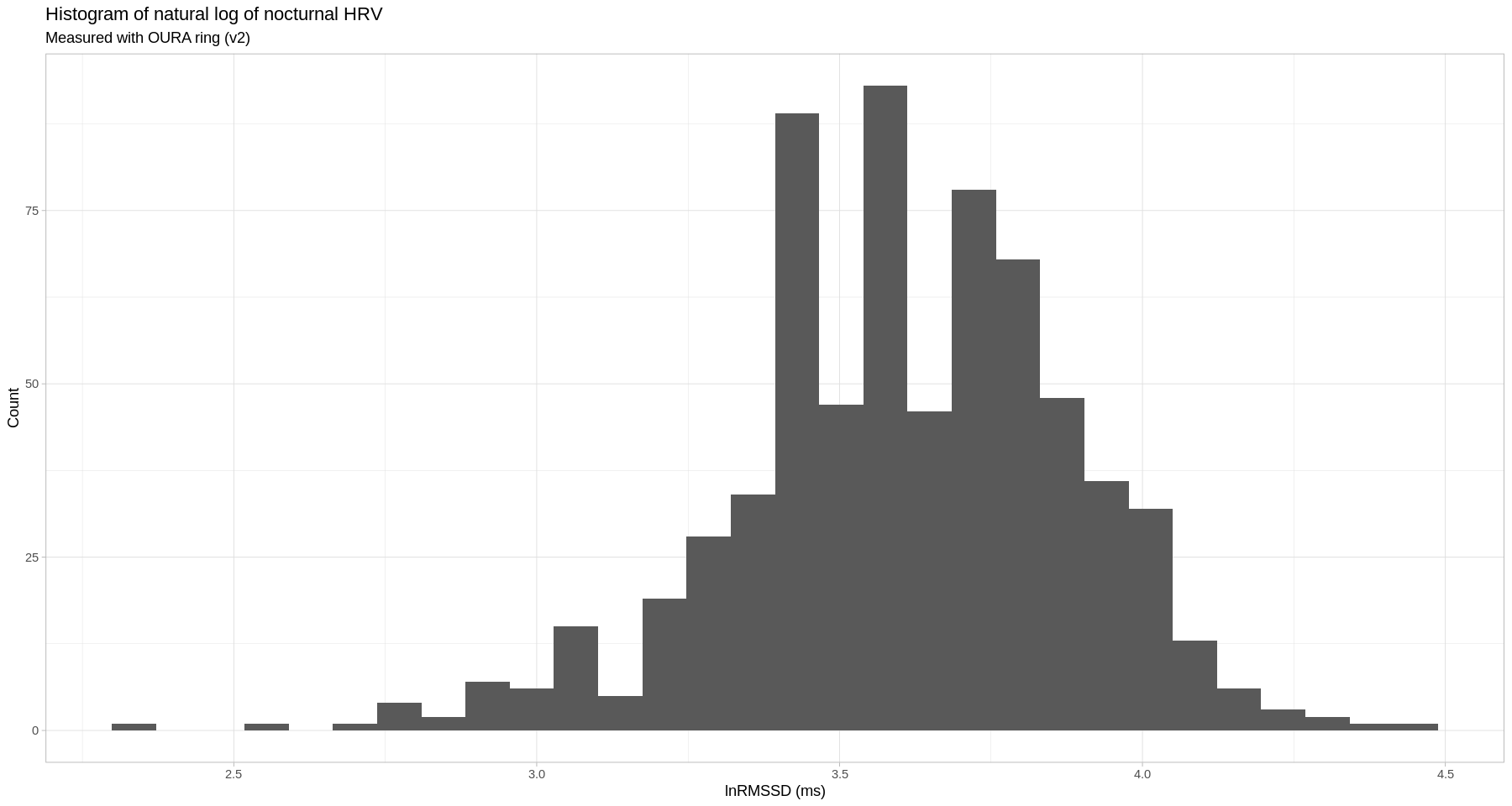```	Shapiro-Wilk normality test

data:  df_oura\$hrv
W = 0.98304, p-value = 3.867e-07
```
```	Shapiro-Wilk normality test

data:  df_oura\$lnhrv
W = 0.98515, p-value = 1.945e-06
```
````stat_bin()` using `bins = 30`. Pick better value with `binwidth`.

```
```	Shapiro-Wilk normality test

data:  df_oura\$tst_hr
W = 0.99591, p-value = 0.06978
```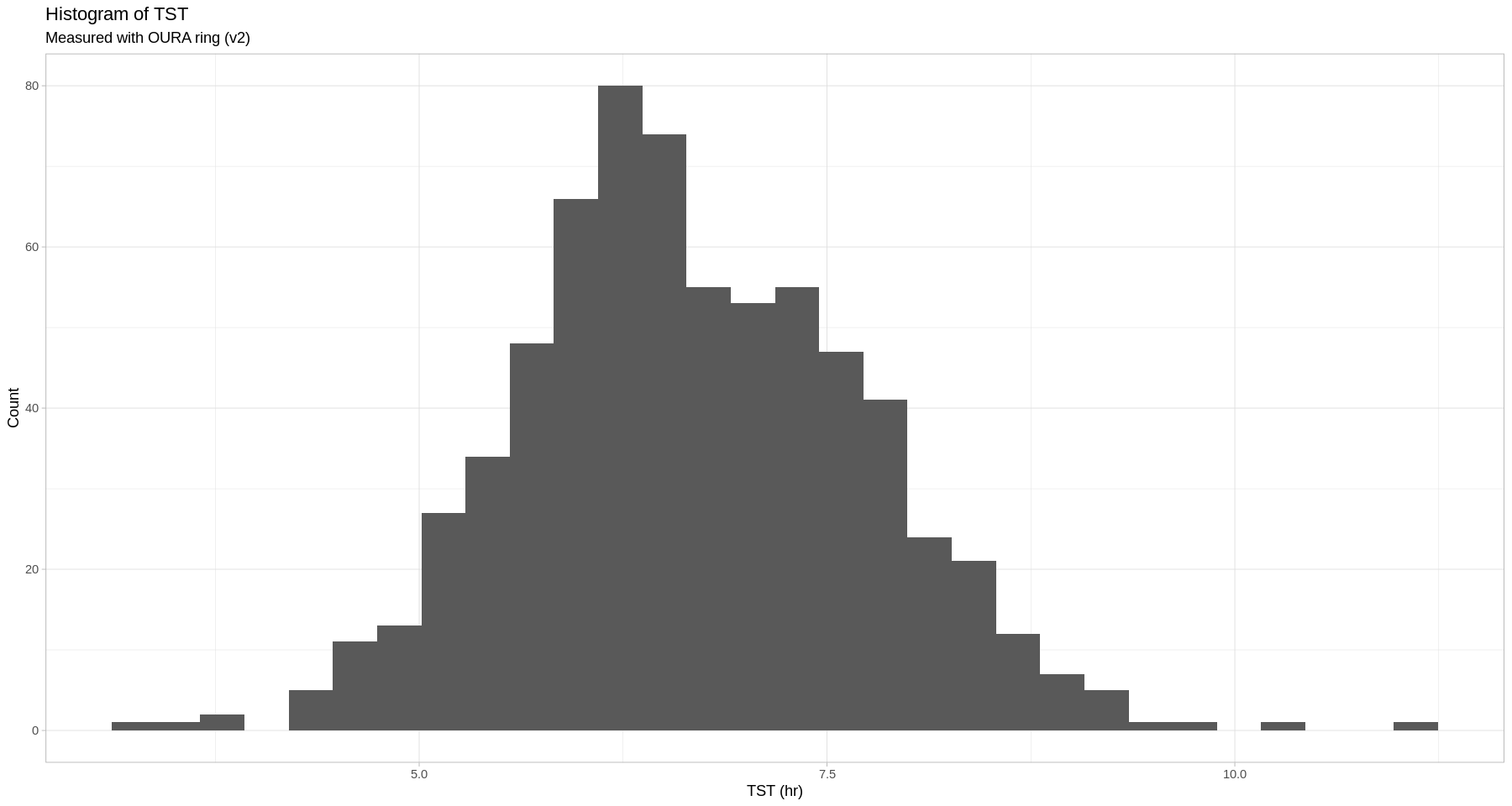## HRV versus physical activity¶

```Updating HTML index of packages in '.Library'

Making 'packages.html' ...
done

```
```Call:
lm(formula = lead(hrv) ~ vig + mod + lig + sed, data = df_oura)

Residuals:
Min      1Q  Median      3Q     Max
-28.644  -6.416  -0.143   6.276  33.682

Coefficients:
Estimate Std. Error t value Pr(>|t|)
(Intercept) 23.346561   2.947085   7.922 9.56e-15 ***
vig          0.003677   0.006083   0.604    0.546
mod          0.014330   0.002558   5.601 3.09e-08 ***
lig          0.050185   0.006174   8.129 2.05e-15 ***
sed          0.005587   0.003750   1.490    0.137
---
Signif. codes:  0 ‘***’ 0.001 ‘**’ 0.01 ‘*’ 0.05 ‘.’ 0.1 ‘ ’ 1

Residual standard error: 9.621 on 680 degrees of freedom
(1 observation deleted due to missingness)
Multiple R-squared:  0.157,	Adjusted R-squared:  0.152
F-statistic: 31.66 on 4 and 680 DF,  p-value: < 2.2e-16
```
```Updating HTML index of packages in '.Library'

Making 'packages.html' ...
done

Updating HTML index of packages in '.Library'

Making 'packages.html' ...
done

`geom_smooth()` using formula 'y ~ x'

`geom_smooth()` using formula 'y ~ x'

`geom_smooth()` using formula 'y ~ x'

`stat_bin()` using `bins = 30`. Pick better value with `binwidth`.

Warning message:
“Removed 685 rows containing missing values (geom_text).”
```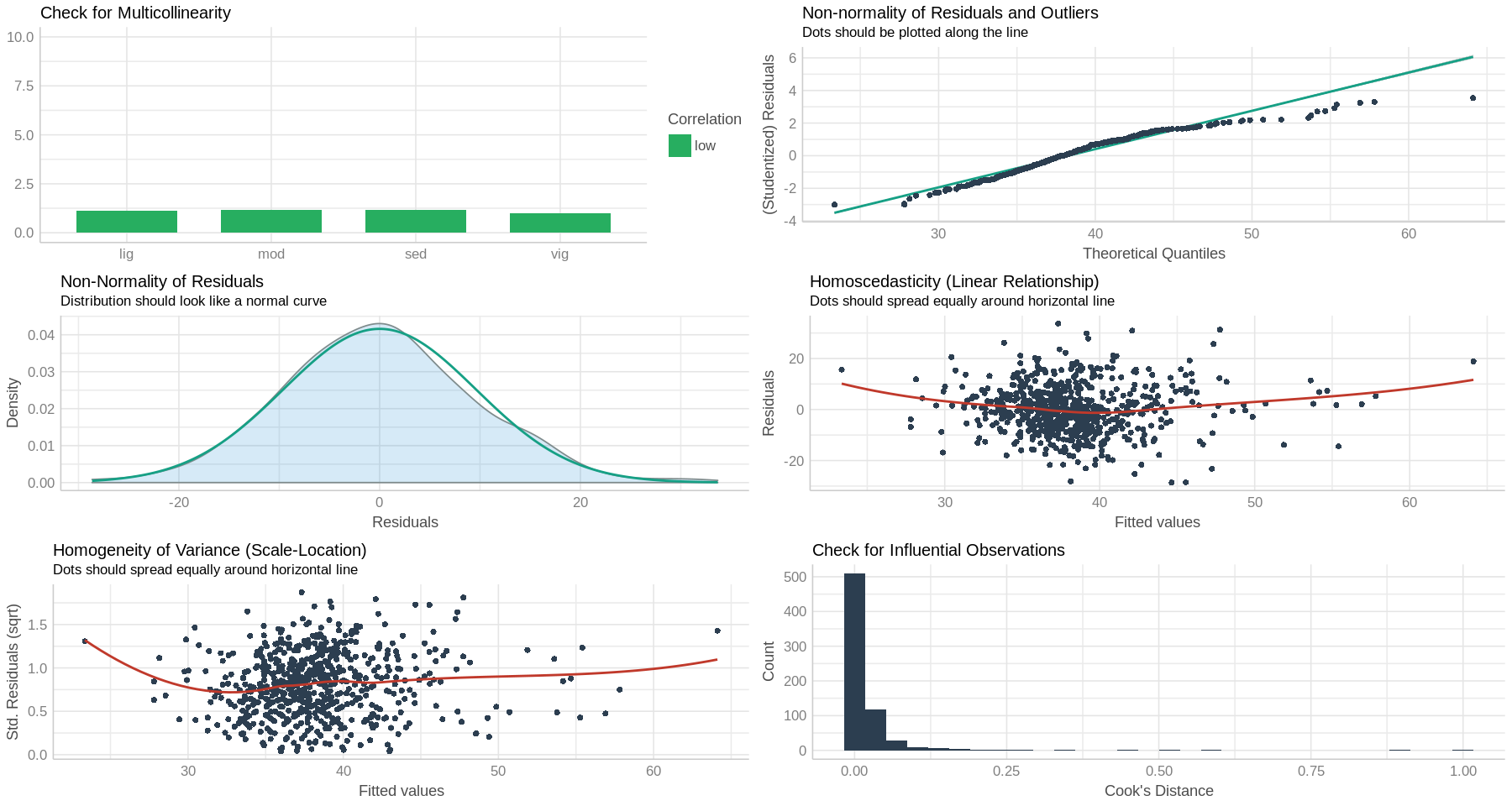```Call:
lm(formula = mvpa ~ hrv, data = df_oura)

Residuals:
Min      1Q  Median      3Q     Max
-301.72 -103.91  -11.79   77.76 1084.15

Coefficients:
Estimate Std. Error t value Pr(>|t|)
(Intercept) 188.9329    23.5265   8.031 4.24e-15 ***
hrv           2.5645     0.5931   4.324 1.76e-05 ***
---
Signif. codes:  0 ‘***’ 0.001 ‘**’ 0.01 ‘*’ 0.05 ‘.’ 0.1 ‘ ’ 1

Residual standard error: 162.1 on 684 degrees of freedom
Multiple R-squared:  0.02661,	Adjusted R-squared:  0.02518
F-statistic:  18.7 on 1 and 684 DF,  p-value: 1.761e-05
```
```Not enough model terms in the conditional part of the model to check for multicollinearity.
```
````geom_smooth()` using formula 'y ~ x'

`geom_smooth()` using formula 'y ~ x'

`geom_smooth()` using formula 'y ~ x'

`stat_bin()` using `bins = 30`. Pick better value with `binwidth`.

Warning message:
“Removed 686 rows containing missing values (geom_text).”
```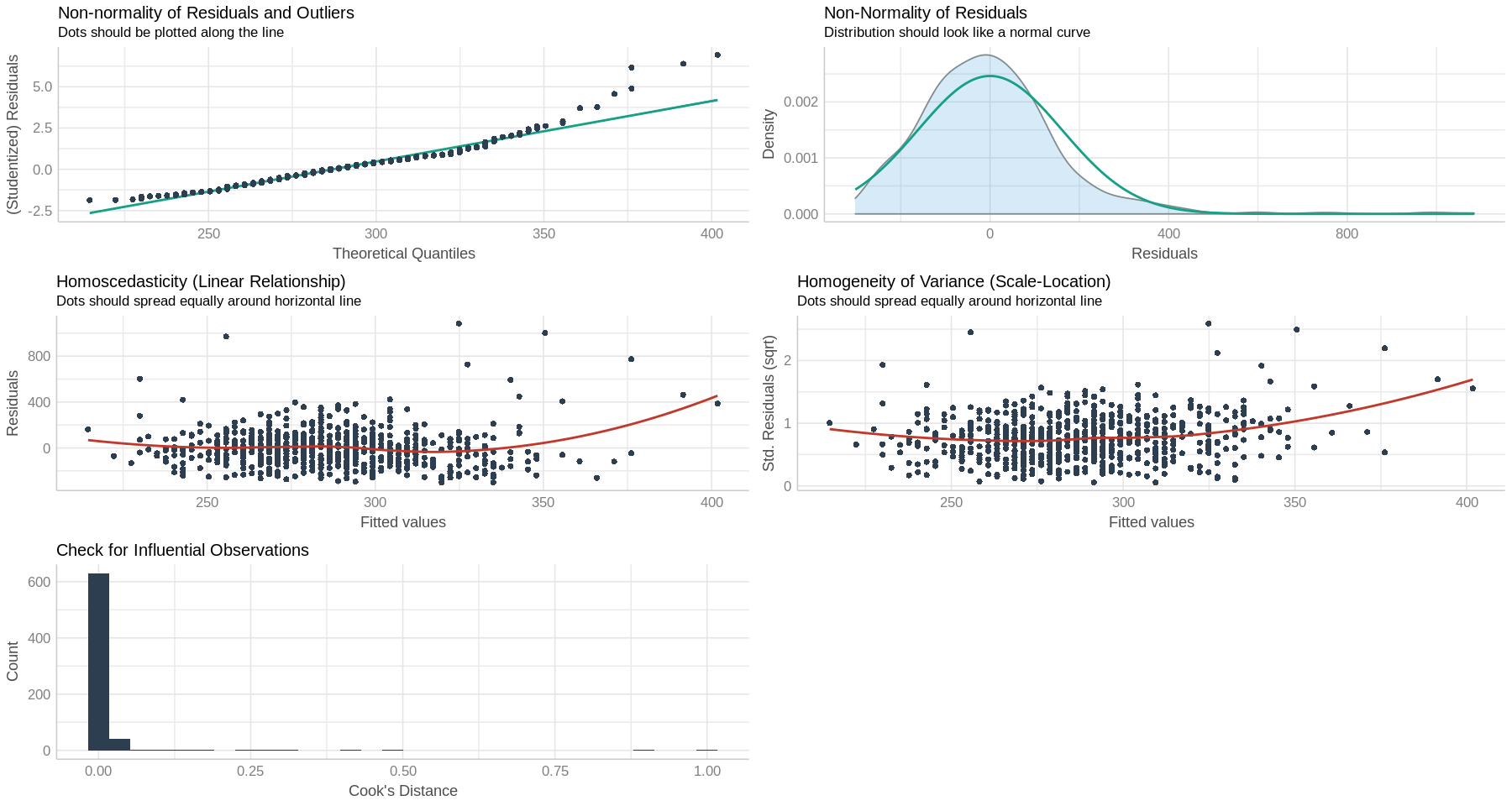```Call:
lm(formula = mvpa ~ lnhrv, data = df_oura)

Residuals:
Min      1Q  Median      3Q     Max
-295.95 -102.46  -13.37   79.55 1091.88

Coefficients:
Estimate Std. Error t value Pr(>|t|)
(Intercept)   -10.69      79.57  -0.134 0.893168
lnhrv          82.57      22.00   3.753 0.000189 ***
---
Signif. codes:  0 ‘***’ 0.001 ‘**’ 0.01 ‘*’ 0.05 ‘.’ 0.1 ‘ ’ 1

Residual standard error: 162.6 on 684 degrees of freedom
Multiple R-squared:  0.02018,	Adjusted R-squared:  0.01875
F-statistic: 14.09 on 1 and 684 DF,  p-value: 0.0001892
```
```Not enough model terms in the conditional part of the model to check for multicollinearity.
```
````geom_smooth()` using formula 'y ~ x'

`geom_smooth()` using formula 'y ~ x'

`geom_smooth()` using formula 'y ~ x'

`stat_bin()` using `bins = 30`. Pick better value with `binwidth`.

Warning message:
“Removed 686 rows containing missing values (geom_text).”
```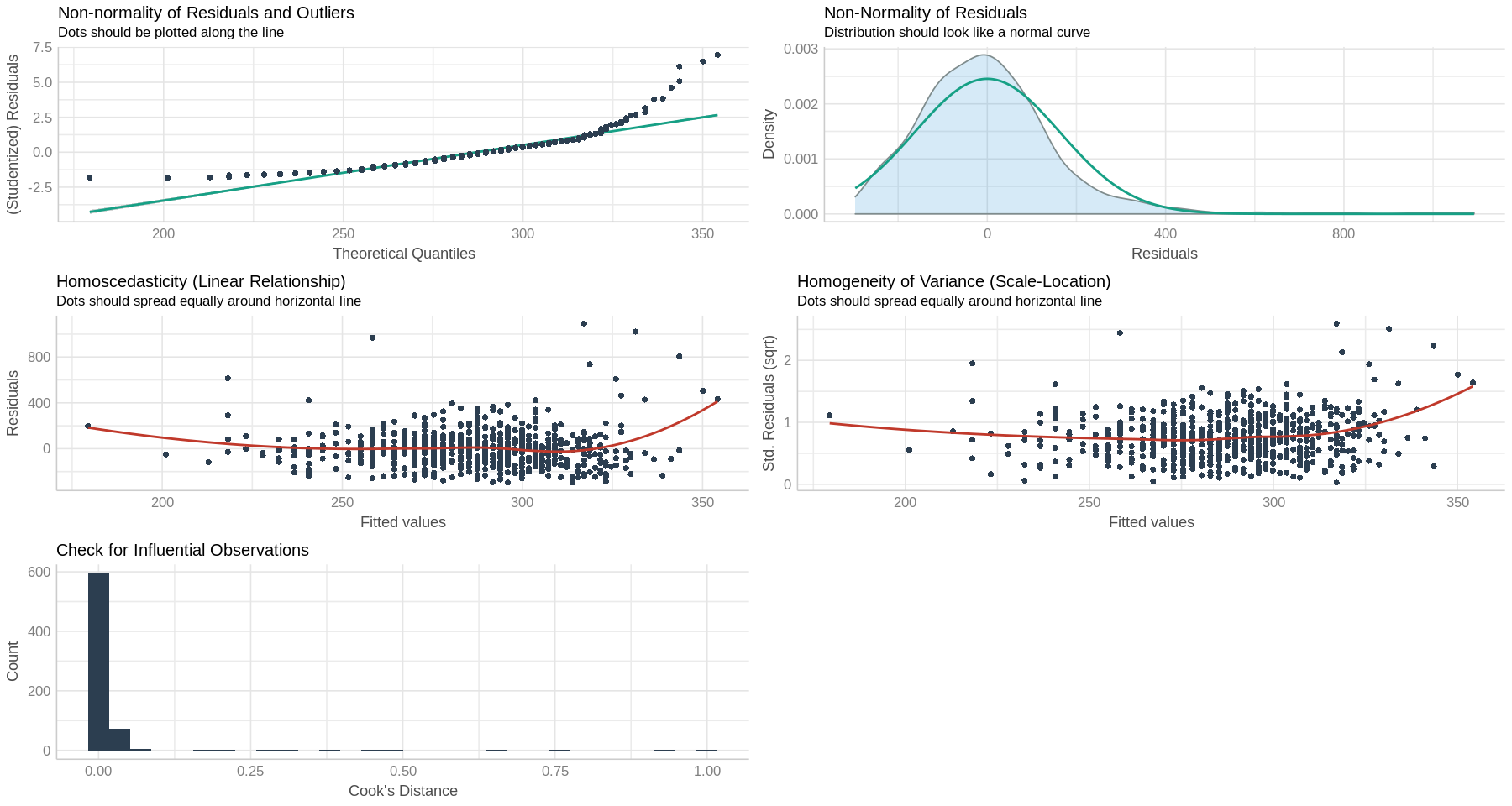```Call:
lm(formula = sed ~ hrv, data = df_oura)

Residuals:
Min      1Q  Median      3Q     Max
-646.24  -62.30    8.82   71.24  298.32

Coefficients:
Estimate Std. Error t value Pr(>|t|)
(Intercept) 715.5453    15.0898  47.419  < 2e-16 ***
hrv          -2.2770     0.3804  -5.986 3.48e-09 ***
---
Signif. codes:  0 ‘***’ 0.001 ‘**’ 0.01 ‘*’ 0.05 ‘.’ 0.1 ‘ ’ 1

Residual standard error: 104 on 684 degrees of freedom
Multiple R-squared:  0.04977,	Adjusted R-squared:  0.04838
F-statistic: 35.83 on 1 and 684 DF,  p-value: 3.477e-09
```
```Not enough model terms in the conditional part of the model to check for multicollinearity.
```
````geom_smooth()` using formula 'y ~ x'

`geom_smooth()` using formula 'y ~ x'

`geom_smooth()` using formula 'y ~ x'

`stat_bin()` using `bins = 30`. Pick better value with `binwidth`.

Warning message:
“Removed 686 rows containing missing values (geom_text).”
```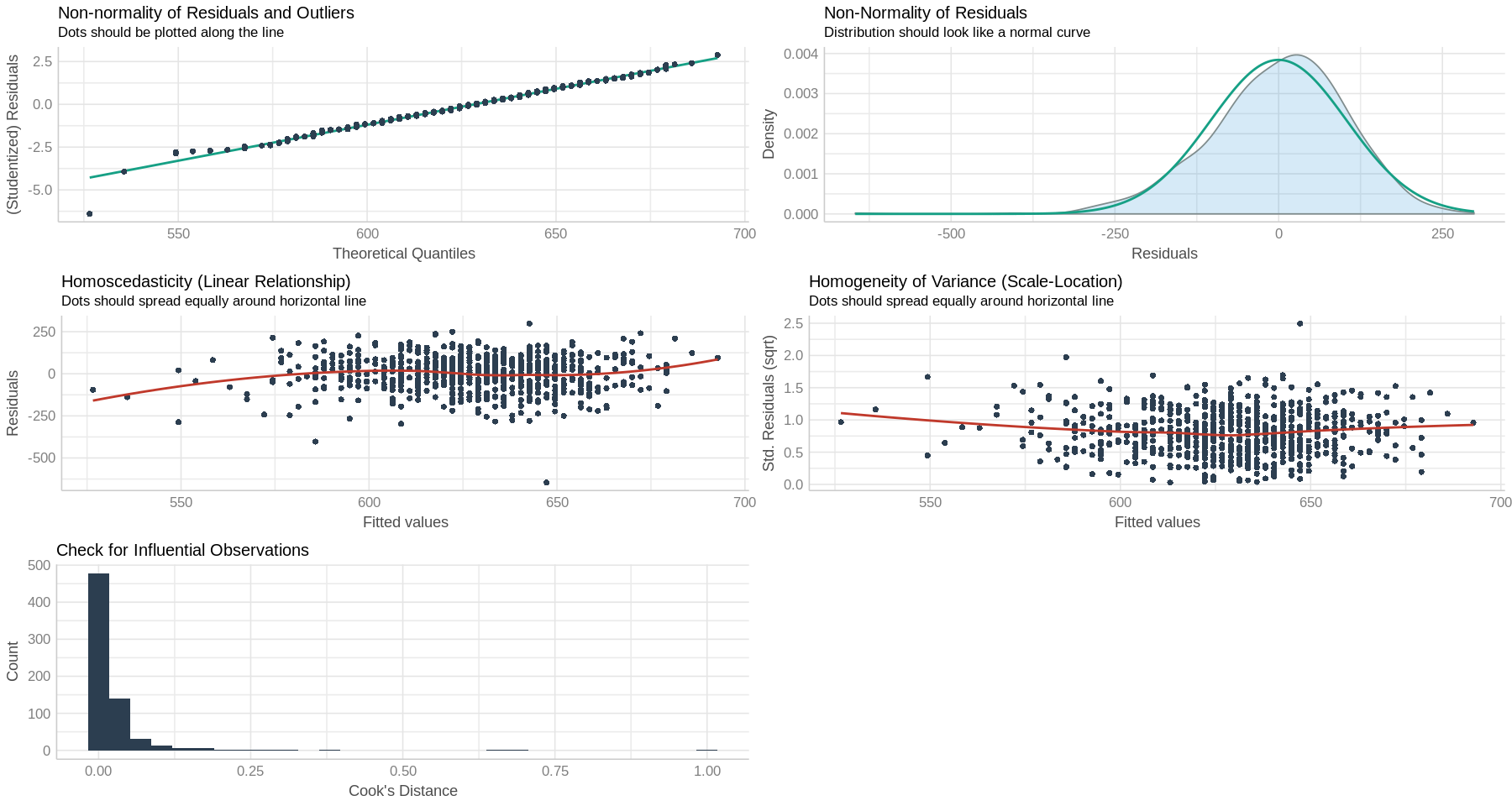```Call:
lm(formula = mvpa ~ hrv_7dra, data = df_oura)

Residuals:
Min      1Q  Median      3Q     Max
-322.87 -104.15  -14.11   74.43 1083.56

Coefficients:
Estimate Std. Error t value Pr(>|t|)
(Intercept) 126.1401    33.2742   3.791 0.000163 ***
hrv_7dra      4.2020     0.8539   4.921 1.08e-06 ***
---
Signif. codes:  0 ‘***’ 0.001 ‘**’ 0.01 ‘*’ 0.05 ‘.’ 0.1 ‘ ’ 1

Residual standard error: 161.9 on 678 degrees of freedom
(6 observations deleted due to missingness)
Multiple R-squared:  0.03449,	Adjusted R-squared:  0.03306
F-statistic: 24.22 on 1 and 678 DF,  p-value: 1.082e-06
```
```Not enough model terms in the conditional part of the model to check for multicollinearity.
```
````geom_smooth()` using formula 'y ~ x'

`geom_smooth()` using formula 'y ~ x'

`geom_smooth()` using formula 'y ~ x'

`stat_bin()` using `bins = 30`. Pick better value with `binwidth`.

Warning message:
“Removed 680 rows containing missing values (geom_text).”
```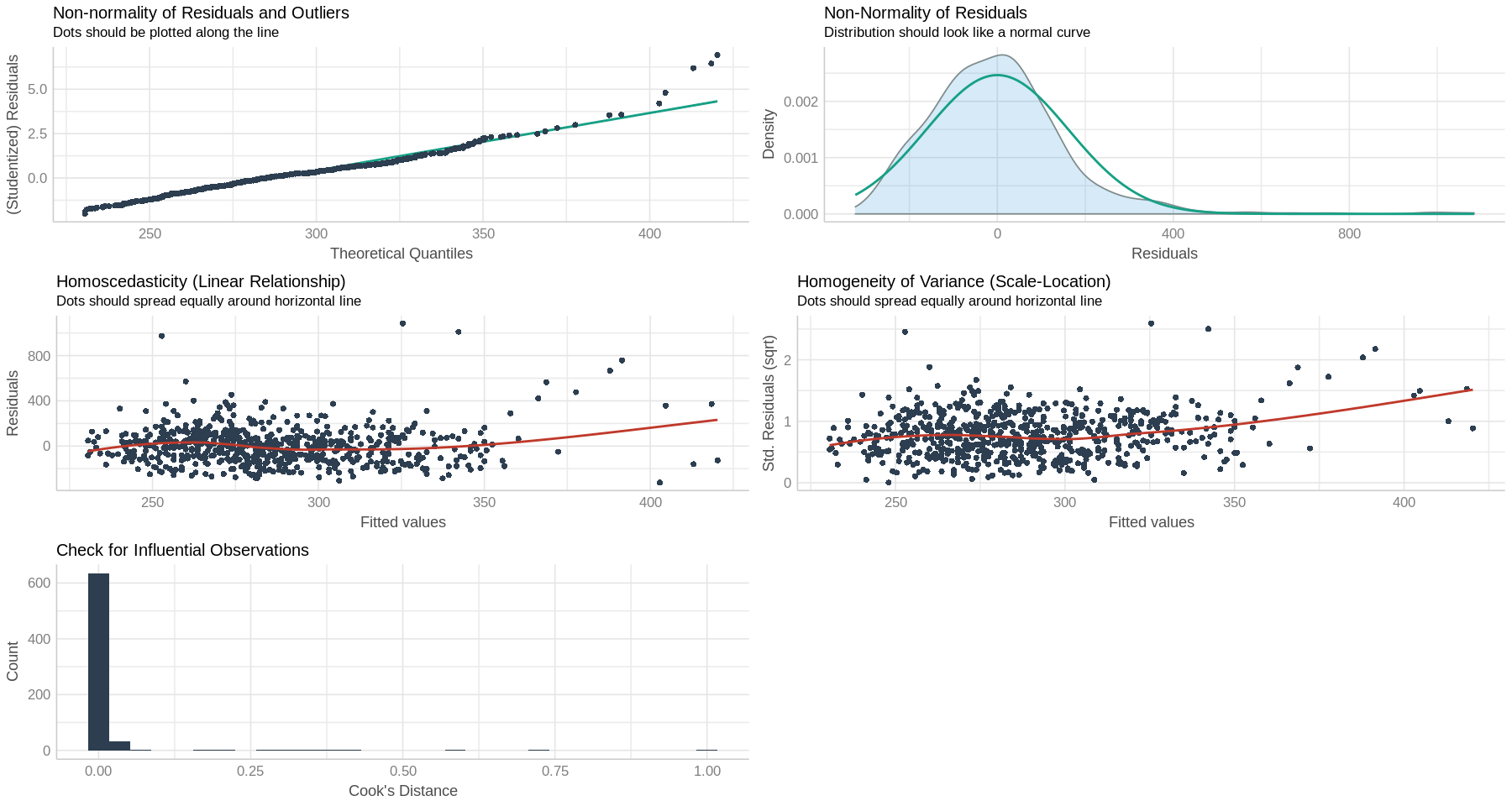```Call:
lm(formula = sed ~ hrv_7dra, data = df_oura)

Residuals:
Min      1Q  Median      3Q     Max
-643.13  -61.16    4.49   71.26  314.27

Coefficients:
Estimate Std. Error t value Pr(>|t|)
(Intercept) 724.3684    21.5777  33.570  < 2e-16 ***
hrv_7dra     -2.4854     0.5537  -4.488 8.43e-06 ***
---
Signif. codes:  0 ‘***’ 0.001 ‘**’ 0.01 ‘*’ 0.05 ‘.’ 0.1 ‘ ’ 1

Residual standard error: 105 on 678 degrees of freedom
(6 observations deleted due to missingness)
Multiple R-squared:  0.02886,	Adjusted R-squared:  0.02742
F-statistic: 20.15 on 1 and 678 DF,  p-value: 8.429e-06
```
```Not enough model terms in the conditional part of the model to check for multicollinearity.
```
````geom_smooth()` using formula 'y ~ x'

`geom_smooth()` using formula 'y ~ x'

`geom_smooth()` using formula 'y ~ x'

`stat_bin()` using `bins = 30`. Pick better value with `binwidth`.

Warning message:
“Removed 680 rows containing missing values (geom_text).”
```